Next: Figure 1 Up: Normal Modes and Soliton Previous: The Model.

# Magnon and Vortex Excitations.

In the absence of vortices, this 2D-model has well-known magnon excitations about the classical AFM ground state. There are two branches of magnons: ``in-plane'' one with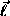oscillating in the easy-plane, and ``out-of-plane'' one withoscillating in the z-direction, with out-of-plane and in-plane oscillations of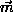, respectively. These have gapless and finite activation dispersion laws, respectively: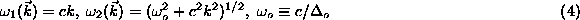where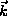is the wave vector. These can be also obtained from a Taylor expansion of the dispersion law for the discrete model, see Ref. .

In the continuum models like Eq. (2), the out-of-plane vortex with the usual topological charges of vorticityand polarization p is described by the solution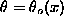,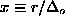,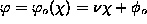, where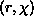are polar coordinates,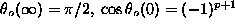, and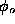is a constant. Only the case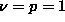will be discussed here. The function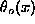is described by an ordinary differential equation (ODE) which can be solved numerically by a shooting method, see ,.

The distribution of spins in the static vortex also can be analyzed directly from the discrete model (1), see ,. Energy minimization is carried out starting from a nearly in-plane structure, and redirecting spins along the effective fields due to the neighbors, iterating until convergence. The coincidence between the data of these approaches is surprisingly good, even for large anisotropy, see Fig. 1. Large discrepancies appear only for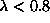\ (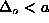), when the vortex structure is rapidly approaching the in-plane form.

Gary M Wysin
Wed Sep 6 18:51:57 CDT 1995